Скачать презентацию Chapter 3 Section 1 Introduction to Vectors Preview

• Количество слайдов: 47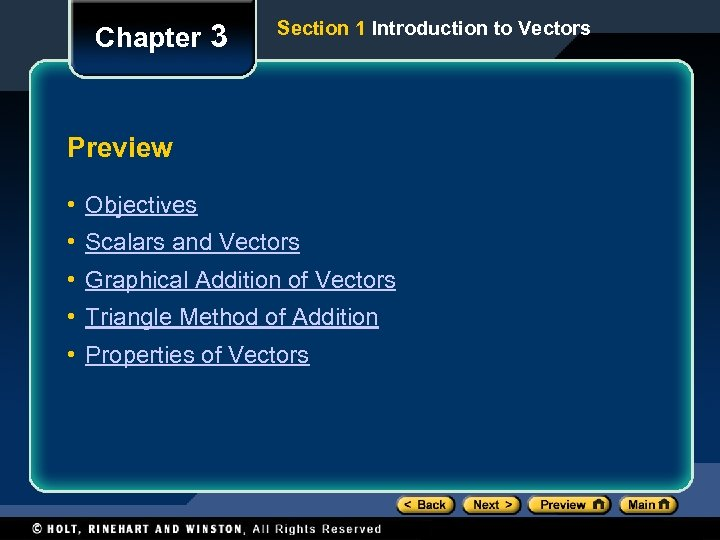Chapter 3 Section 1 Introduction to Vectors Preview • Objectives • Scalars and Vectors • Graphical Addition of Vectors • Triangle Method of Addition • Properties of Vectors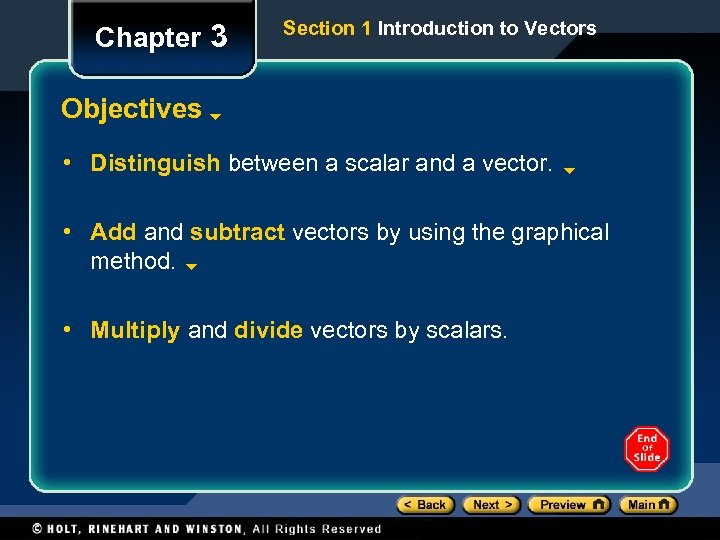Chapter 3 Section 1 Introduction to Vectors Objectives • Distinguish between a scalar and a vector. • Add and subtract vectors by using the graphical method. • Multiply and divide vectors by scalars.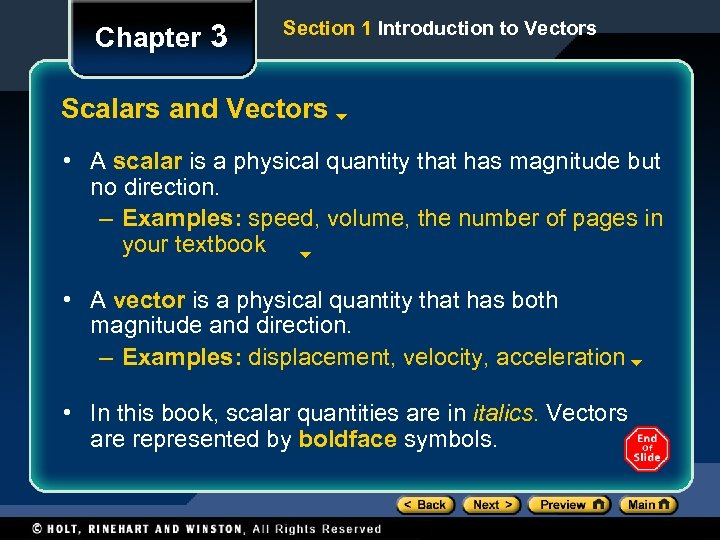Chapter 3 Section 1 Introduction to Vectors Scalars and Vectors • A scalar is a physical quantity that has magnitude but no direction. – Examples: speed, volume, the number of pages in your textbook • A vector is a physical quantity that has both magnitude and direction. – Examples: displacement, velocity, acceleration • In this book, scalar quantities are in italics. Vectors are represented by boldface symbols.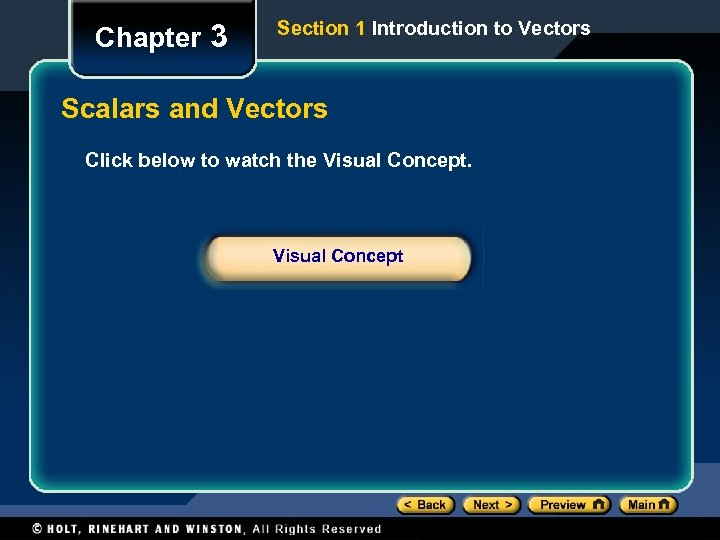Chapter 3 Section 1 Introduction to Vectors Scalars and Vectors Click below to watch the Visual Concept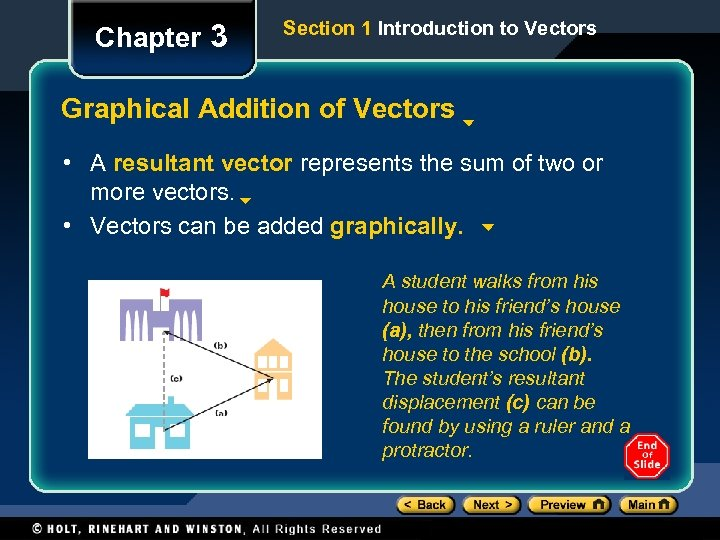Chapter 3 Section 1 Introduction to Vectors Graphical Addition of Vectors • A resultant vector represents the sum of two or more vectors. • Vectors can be added graphically. A student walks from his house to his friend’s house (a), then from his friend’s house to the school (b). The student’s resultant displacement (c) can be found by using a ruler and a protractor.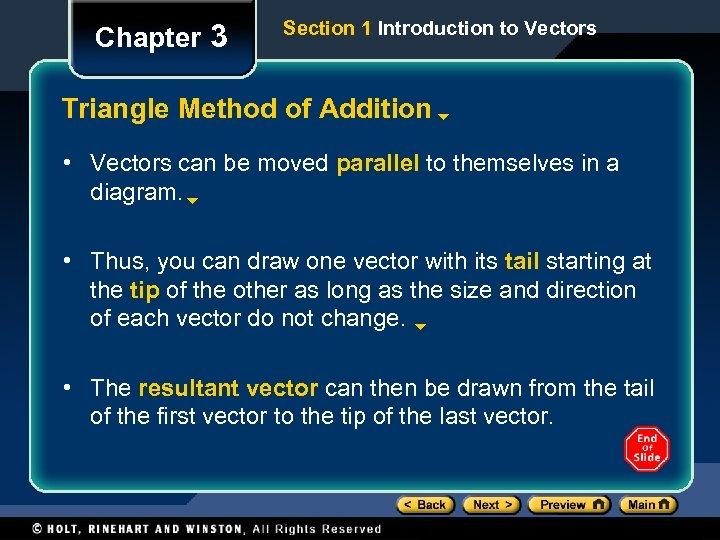Chapter 3 Section 1 Introduction to Vectors Triangle Method of Addition • Vectors can be moved parallel to themselves in a diagram. • Thus, you can draw one vector with its tail starting at the tip of the other as long as the size and direction of each vector do not change. • The resultant vector can then be drawn from the tail of the first vector to the tip of the last vector.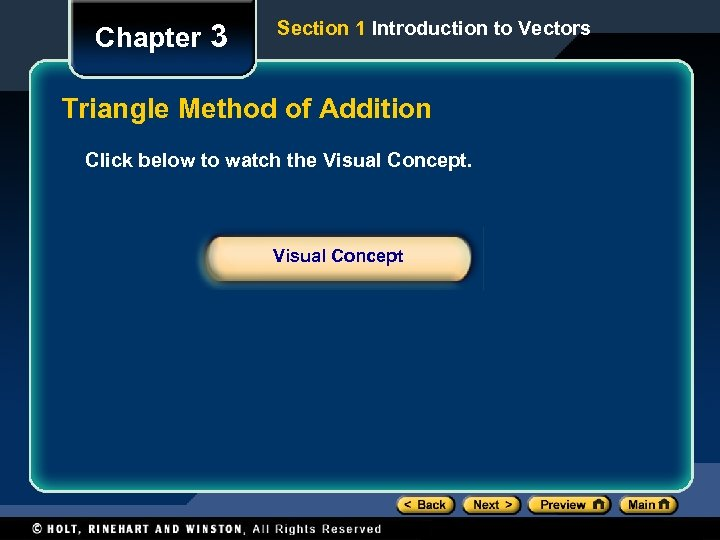Chapter 3 Section 1 Introduction to Vectors Triangle Method of Addition Click below to watch the Visual Concept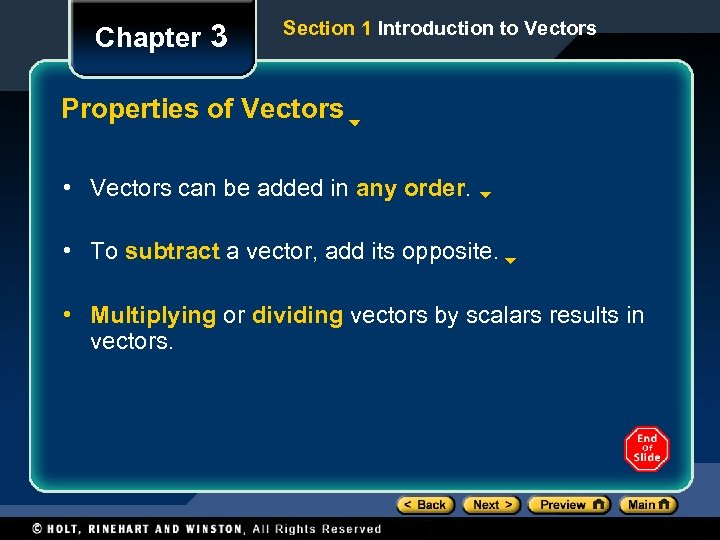Chapter 3 Section 1 Introduction to Vectors Properties of Vectors • Vectors can be added in any order. • To subtract a vector, add its opposite. • Multiplying or dividing vectors by scalars results in vectors.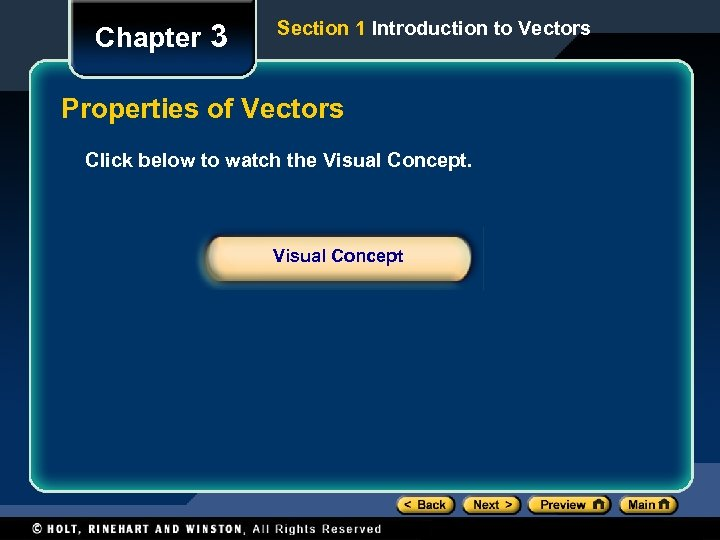Chapter 3 Section 1 Introduction to Vectors Properties of Vectors Click below to watch the Visual Concept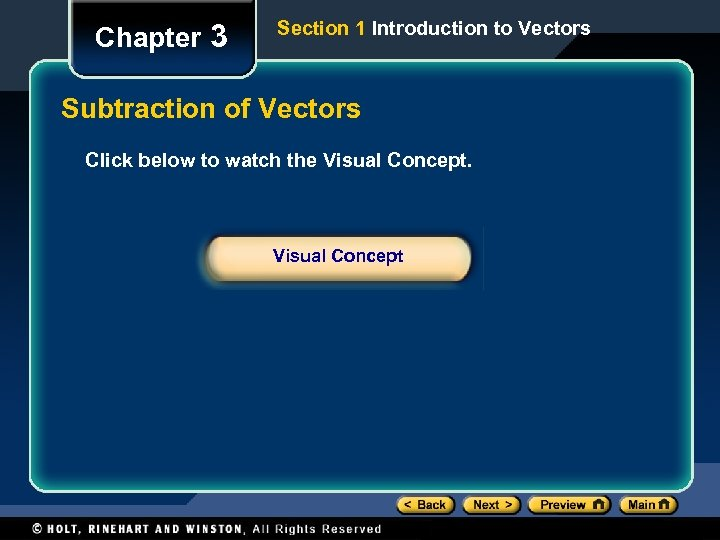Chapter 3 Section 1 Introduction to Vectors Subtraction of Vectors Click below to watch the Visual Concept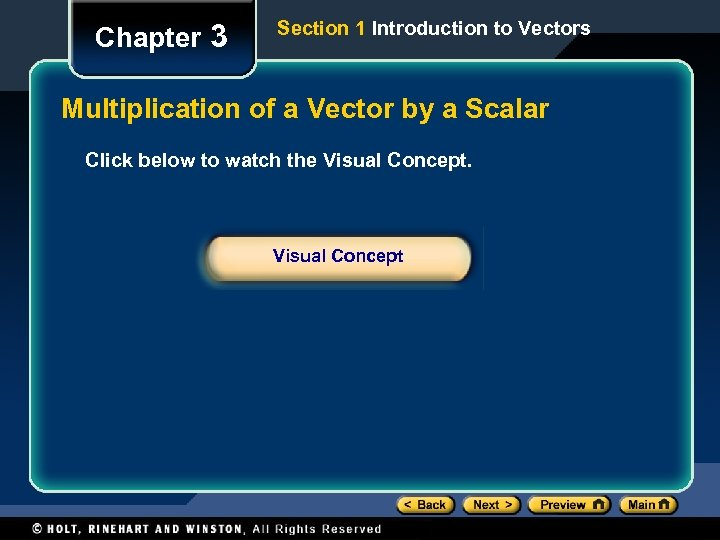Chapter 3 Section 1 Introduction to Vectors Multiplication of a Vector by a Scalar Click below to watch the Visual Concept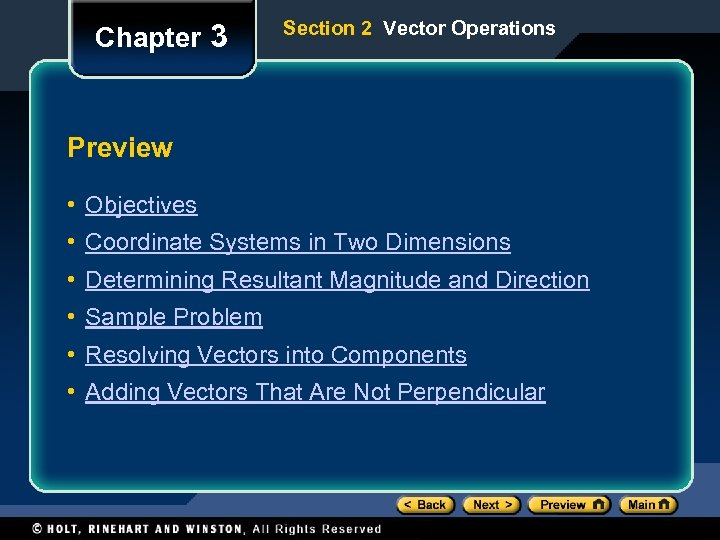Chapter 3 Section 2 Vector Operations Preview • Objectives • Coordinate Systems in Two Dimensions • Determining Resultant Magnitude and Direction • Sample Problem • Resolving Vectors into Components • Adding Vectors That Are Not Perpendicular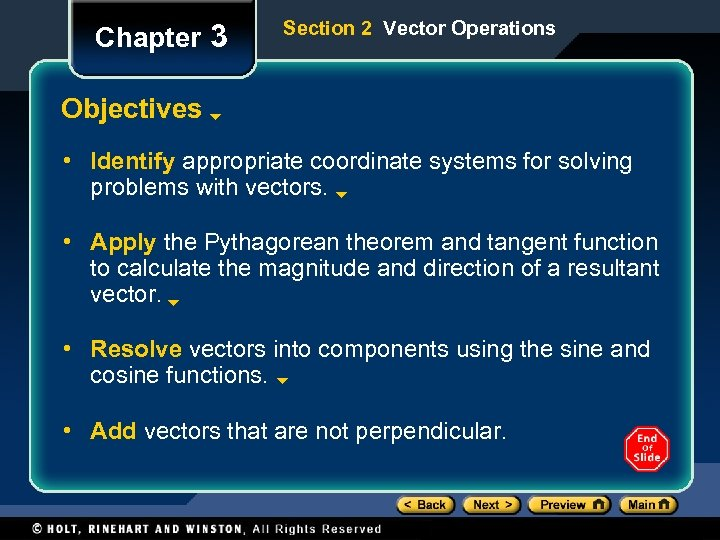Chapter 3 Section 2 Vector Operations Objectives • Identify appropriate coordinate systems for solving problems with vectors. • Apply the Pythagorean theorem and tangent function to calculate the magnitude and direction of a resultant vector. • Resolve vectors into components using the sine and cosine functions. • Add vectors that are not perpendicular.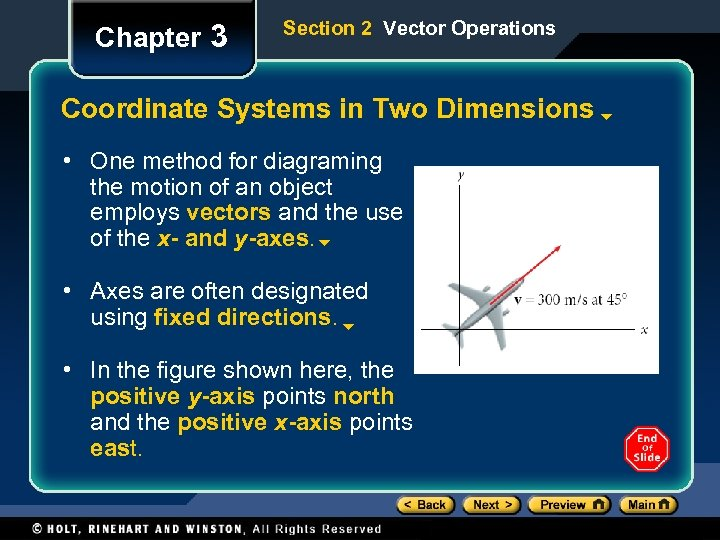Chapter 3 Section 2 Vector Operations Coordinate Systems in Two Dimensions • One method for diagraming the motion of an object employs vectors and the use of the x- and y-axes. • Axes are often designated using fixed directions. • In the figure shown here, the positive y-axis points north and the positive x-axis points east.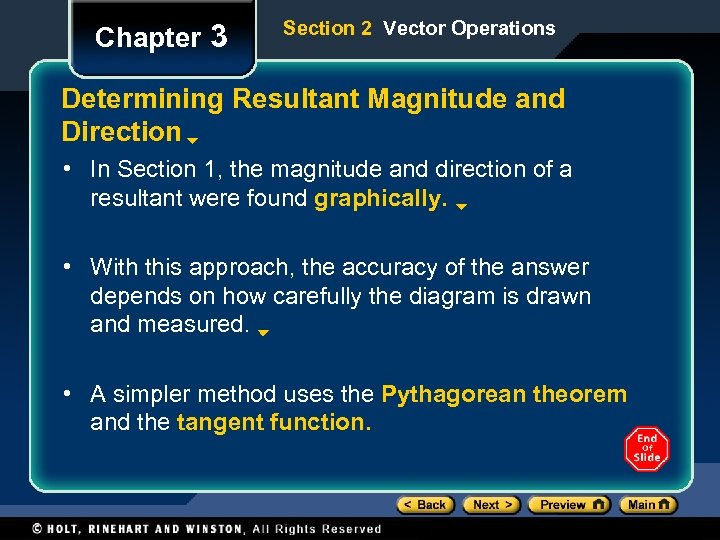Chapter 3 Section 2 Vector Operations Determining Resultant Magnitude and Direction • In Section 1, the magnitude and direction of a resultant were found graphically. • With this approach, the accuracy of the answer depends on how carefully the diagram is drawn and measured. • A simpler method uses the Pythagorean theorem and the tangent function.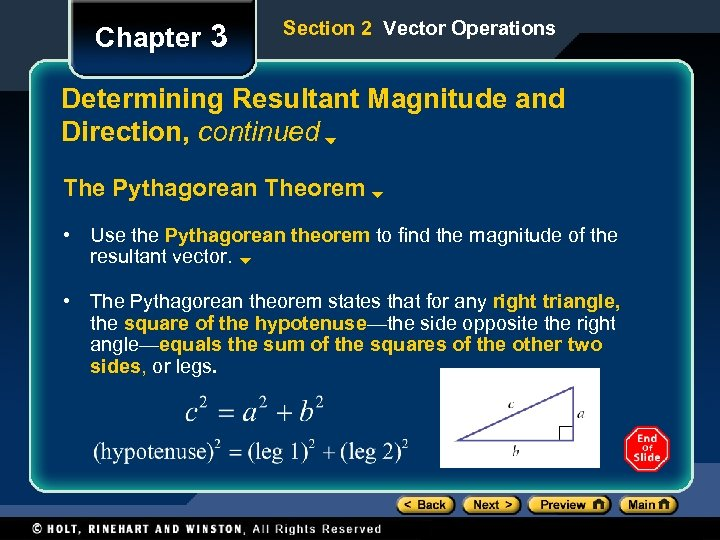Chapter 3 Section 2 Vector Operations Determining Resultant Magnitude and Direction, continued The Pythagorean Theorem • Use the Pythagorean theorem to find the magnitude of the resultant vector. • The Pythagorean theorem states that for any right triangle, the square of the hypotenuse—the side opposite the right angle—equals the sum of the squares of the other two sides, or legs.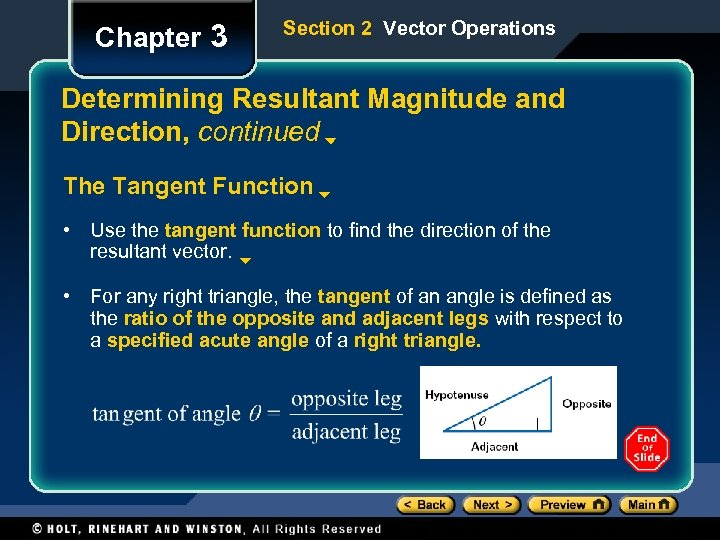Chapter 3 Section 2 Vector Operations Determining Resultant Magnitude and Direction, continued The Tangent Function • Use the tangent function to find the direction of the resultant vector. • For any right triangle, the tangent of an angle is defined as the ratio of the opposite and adjacent legs with respect to a specified acute angle of a right triangle.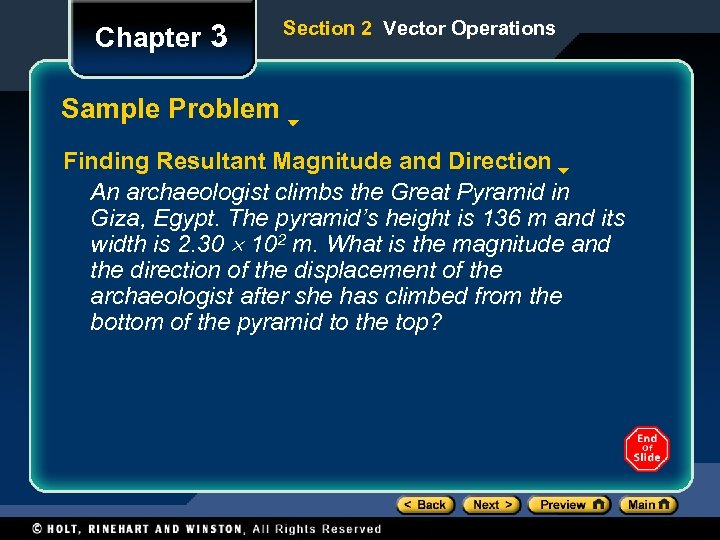Chapter 3 Section 2 Vector Operations Sample Problem Finding Resultant Magnitude and Direction An archaeologist climbs the Great Pyramid in Giza, Egypt. The pyramid’s height is 136 m and its width is 2. 30 102 m. What is the magnitude and the direction of the displacement of the archaeologist after she has climbed from the bottom of the pyramid to the top?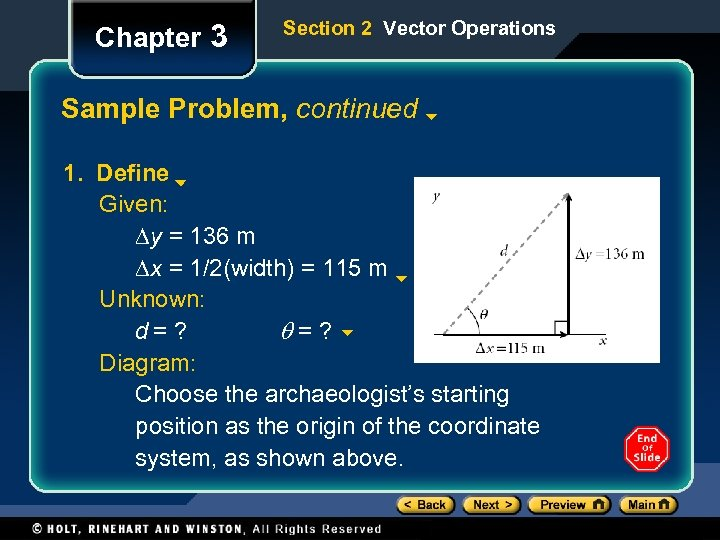Chapter 3 Section 2 Vector Operations Sample Problem, continued 1. Define Given: Dy = 136 m Dx = 1/2(width) = 115 m Unknown: d= ? q=? Diagram: Choose the archaeologist’s starting position as the origin of the coordinate system, as shown above.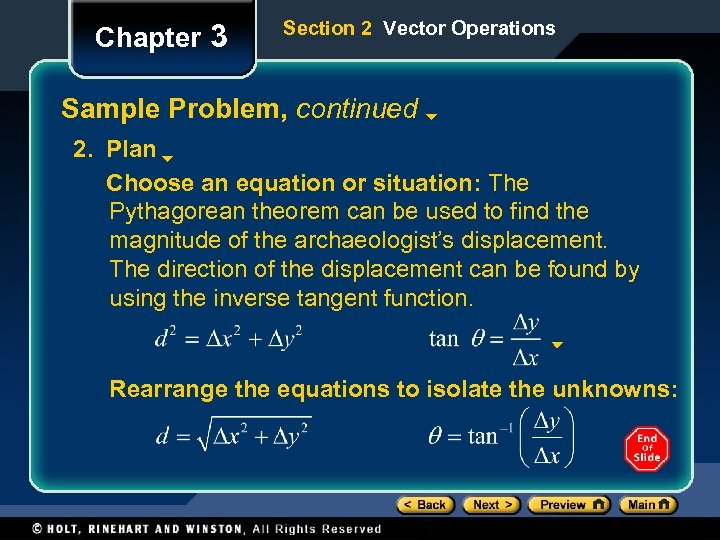Chapter 3 Section 2 Vector Operations Sample Problem, continued 2. Plan Choose an equation or situation: The Pythagorean theorem can be used to find the magnitude of the archaeologist’s displacement. The direction of the displacement can be found by using the inverse tangent function. Rearrange the equations to isolate the unknowns: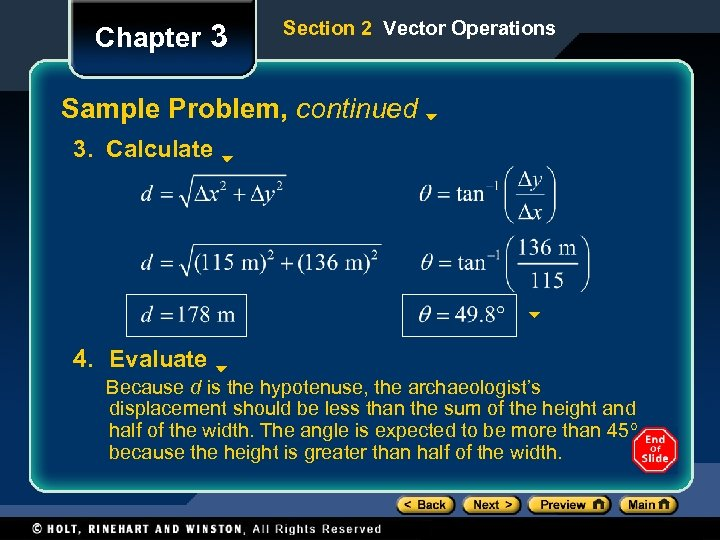Chapter 3 Section 2 Vector Operations Sample Problem, continued 3. Calculate 4. Evaluate Because d is the hypotenuse, the archaeologist’s displacement should be less than the sum of the height and half of the width. The angle is expected to be more than 45 because the height is greater than half of the width.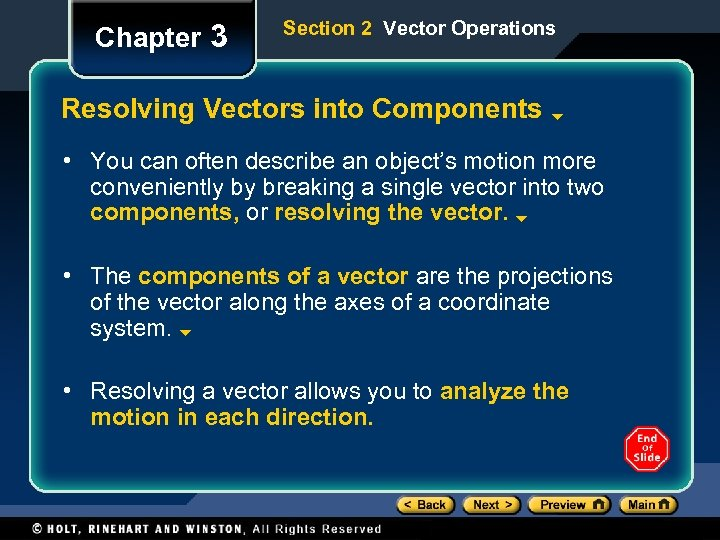Chapter 3 Section 2 Vector Operations Resolving Vectors into Components • You can often describe an object’s motion more conveniently by breaking a single vector into two components, or resolving the vector. • The components of a vector are the projections of the vector along the axes of a coordinate system. • Resolving a vector allows you to analyze the motion in each direction.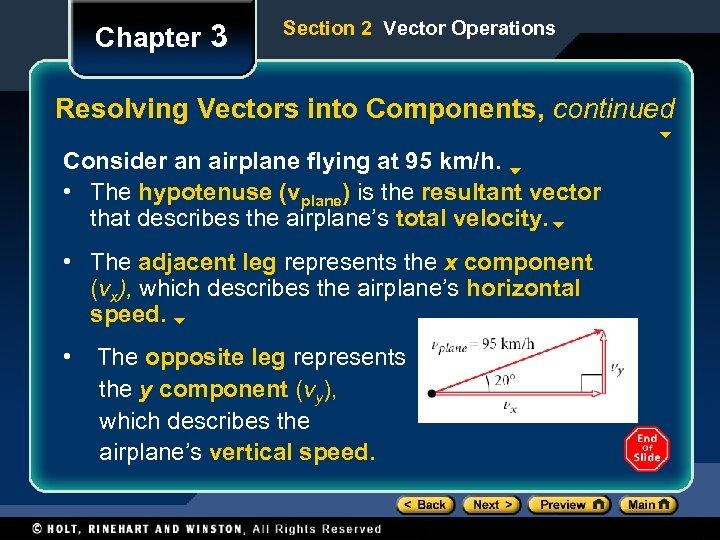Chapter 3 Section 2 Vector Operations Resolving Vectors into Components, continued Consider an airplane flying at 95 km/h. • The hypotenuse (vplane) is the resultant vector that describes the airplane’s total velocity. • The adjacent leg represents the x component (vx), which describes the airplane’s horizontal speed. • The opposite leg represents the y component (vy), which describes the airplane’s vertical speed.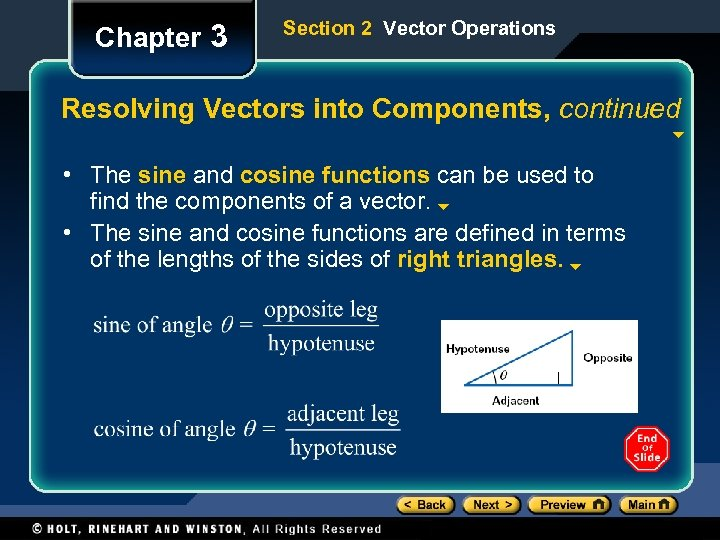Chapter 3 Section 2 Vector Operations Resolving Vectors into Components, continued • The sine and cosine functions can be used to find the components of a vector. • The sine and cosine functions are defined in terms of the lengths of the sides of right triangles.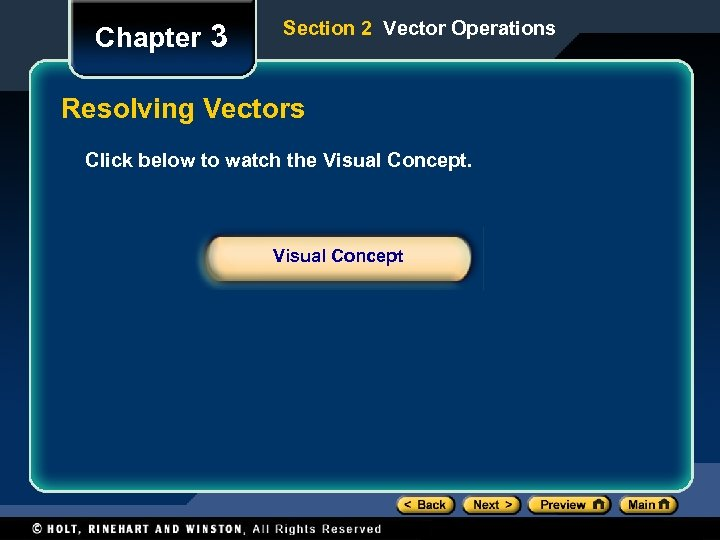Chapter 3 Section 2 Vector Operations Resolving Vectors Click below to watch the Visual Concept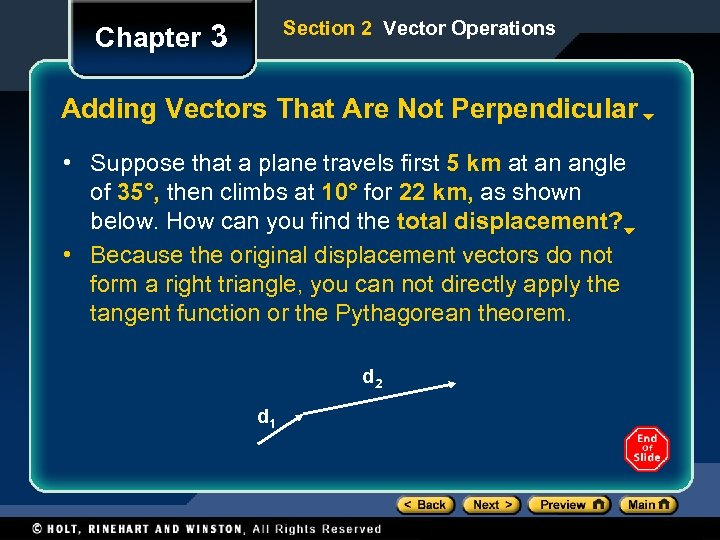Section 2 Vector Operations Chapter 3 Adding Vectors That Are Not Perpendicular • Suppose that a plane travels first 5 km at an angle of 35°, then climbs at 10° for 22 km, as shown below. How can you find the total displacement? • Because the original displacement vectors do not form a right triangle, you can not directly apply the tangent function or the Pythagorean theorem. d 2 d 1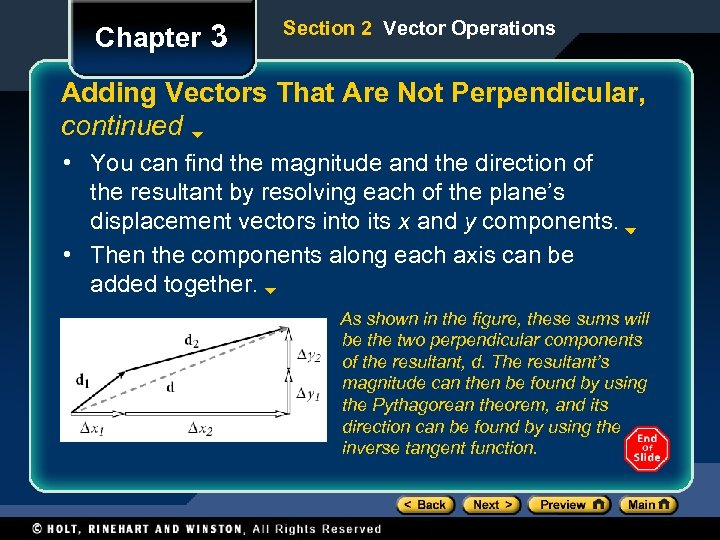Chapter 3 Section 2 Vector Operations Adding Vectors That Are Not Perpendicular, continued • You can find the magnitude and the direction of the resultant by resolving each of the plane’s displacement vectors into its x and y components. • Then the components along each axis can be added together. As shown in the figure, these sums will be the two perpendicular components of the resultant, d. The resultant’s magnitude can then be found by using the Pythagorean theorem, and its direction can be found by using the inverse tangent function.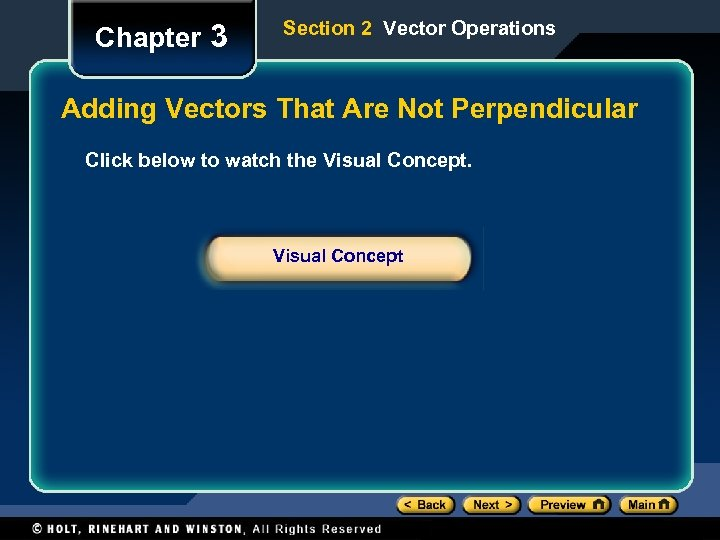Chapter 3 Section 2 Vector Operations Adding Vectors That Are Not Perpendicular Click below to watch the Visual Concept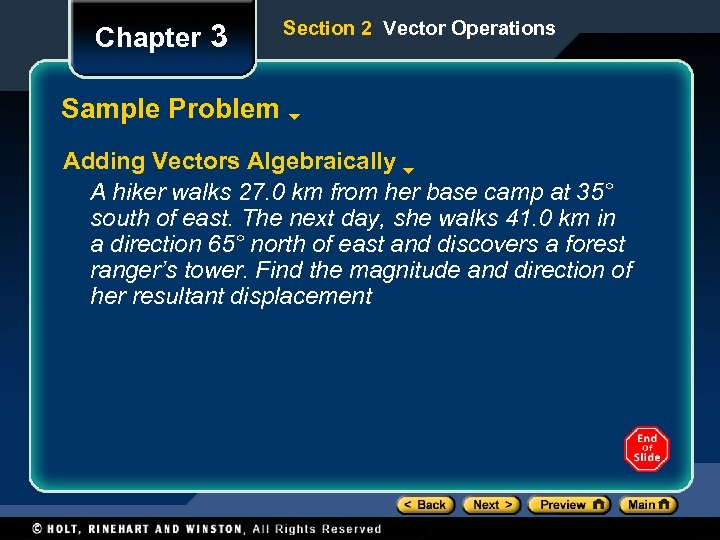Chapter 3 Section 2 Vector Operations Sample Problem Adding Vectors Algebraically A hiker walks 27. 0 km from her base camp at 35° south of east. The next day, she walks 41. 0 km in a direction 65° north of east and discovers a forest ranger’s tower. Find the magnitude and direction of her resultant displacement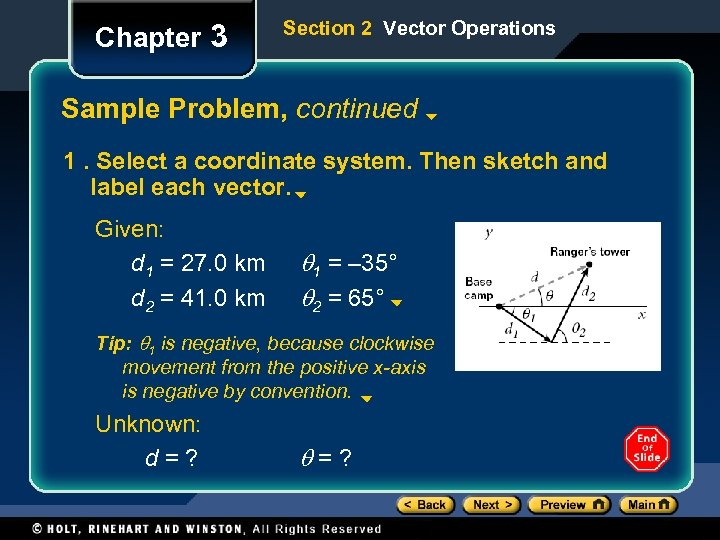Chapter 3 Section 2 Vector Operations Sample Problem, continued 1. Select a coordinate system. Then sketch and label each vector. Given: d 1 = 27. 0 km d 2 = 41. 0 km q 1 = – 35° q 2 = 65° Tip: q 1 is negative, because clockwise movement from the positive x-axis is negative by convention. Unknown: d=? q=?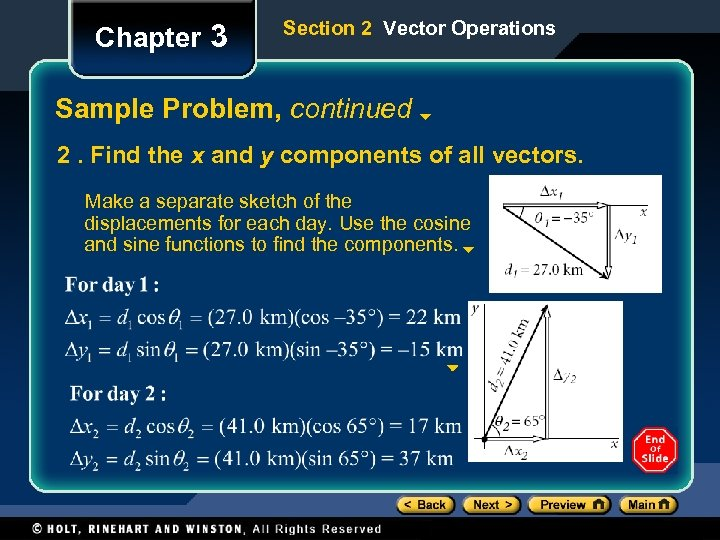Chapter 3 Section 2 Vector Operations Sample Problem, continued 2. Find the x and y components of all vectors. Make a separate sketch of the displacements for each day. Use the cosine and sine functions to find the components.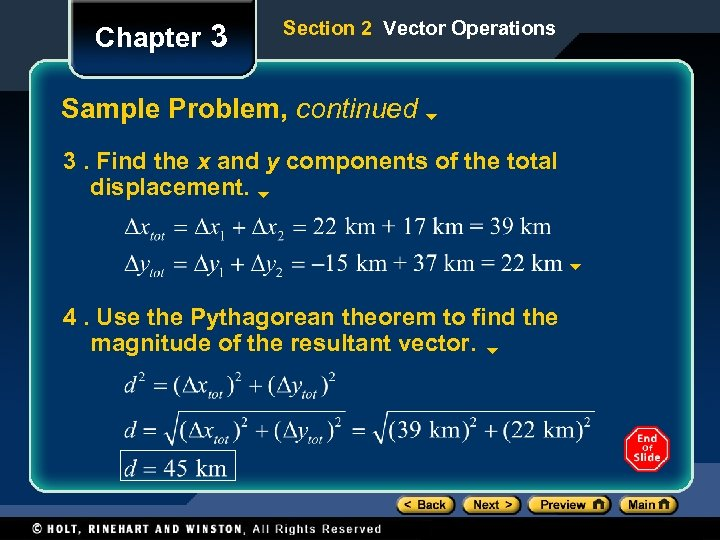Chapter 3 Section 2 Vector Operations Sample Problem, continued 3. Find the x and y components of the total displacement. 4. Use the Pythagorean theorem to find the magnitude of the resultant vector.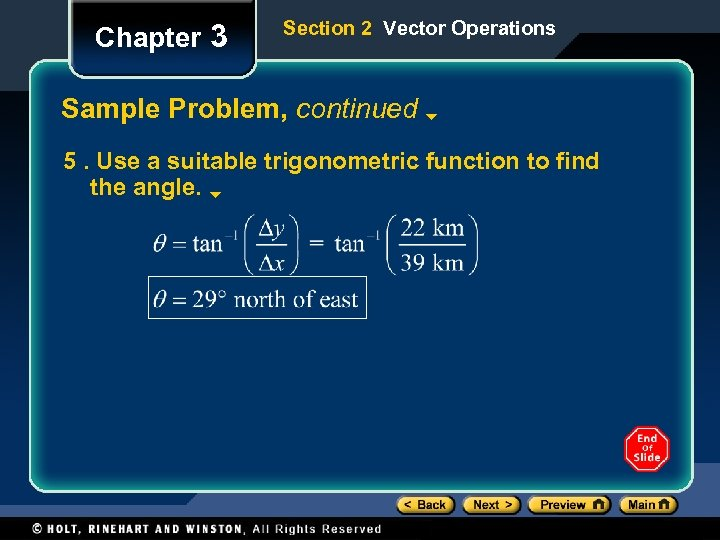Chapter 3 Section 2 Vector Operations Sample Problem, continued 5. Use a suitable trigonometric function to find the angle.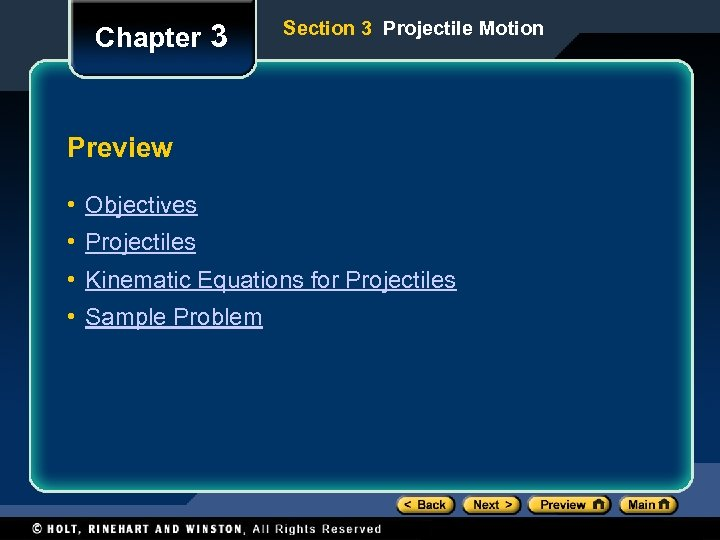Chapter 3 Section 3 Projectile Motion Preview • Objectives • Projectiles • Kinematic Equations for Projectiles • Sample Problem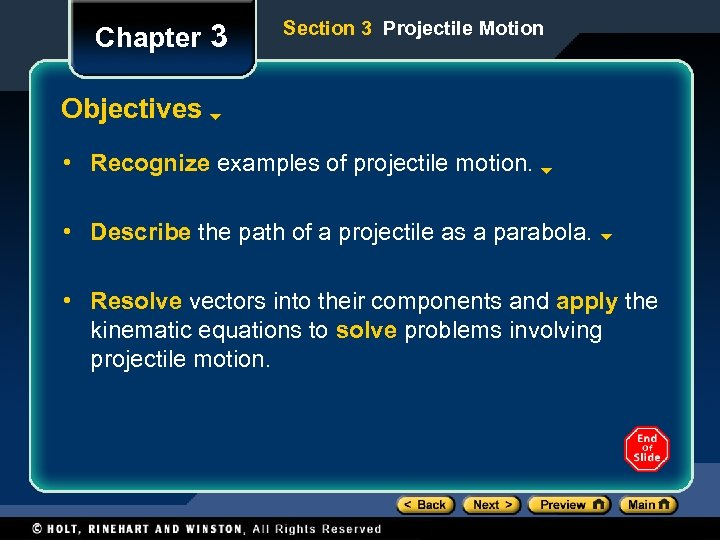Chapter 3 Section 3 Projectile Motion Objectives • Recognize examples of projectile motion. • Describe the path of a projectile as a parabola. • Resolve vectors into their components and apply the kinematic equations to solve problems involving projectile motion.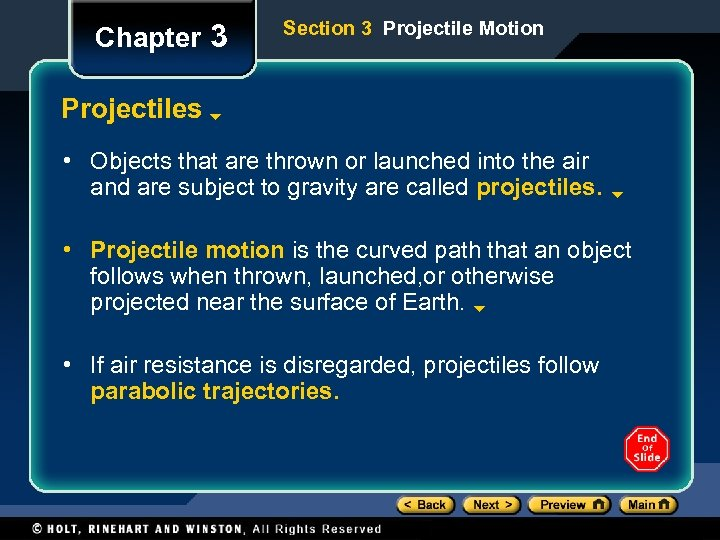Chapter 3 Section 3 Projectile Motion Projectiles • Objects that are thrown or launched into the air and are subject to gravity are called projectiles. • Projectile motion is the curved path that an object follows when thrown, launched, or otherwise projected near the surface of Earth. • If air resistance is disregarded, projectiles follow parabolic trajectories.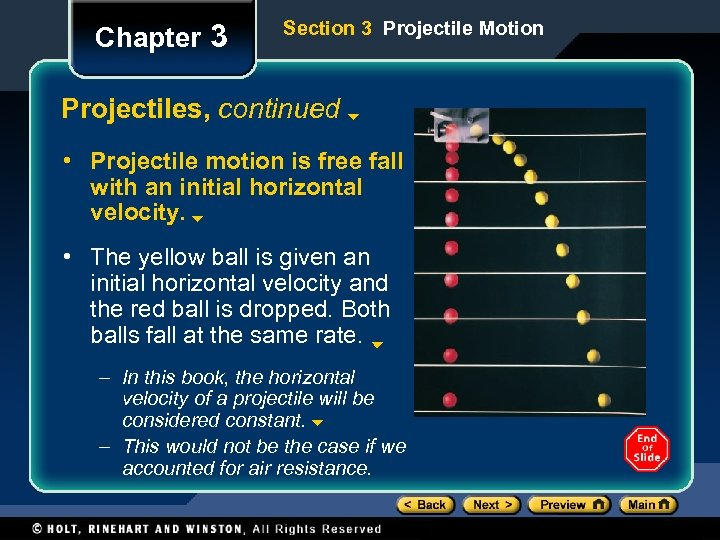Chapter 3 Section 3 Projectile Motion Projectiles, continued • Projectile motion is free fall with an initial horizontal velocity. • The yellow ball is given an initial horizontal velocity and the red ball is dropped. Both balls fall at the same rate. – In this book, the horizontal velocity of a projectile will be considered constant. – This would not be the case if we accounted for air resistance.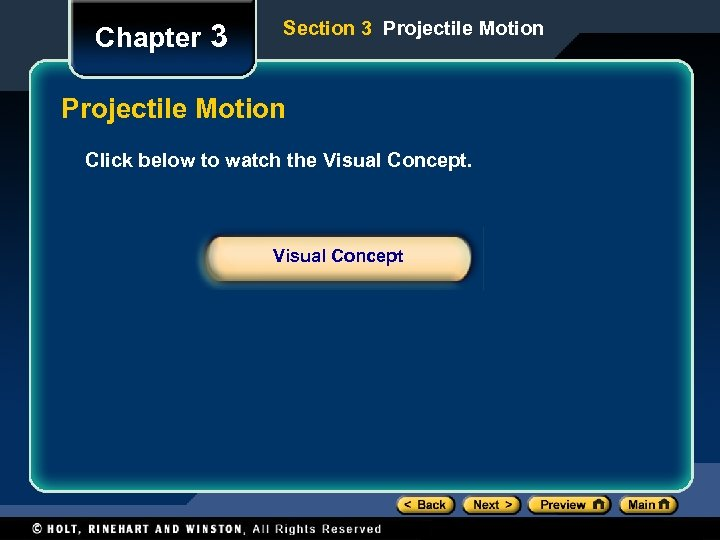Chapter 3 Section 3 Projectile Motion Click below to watch the Visual Concept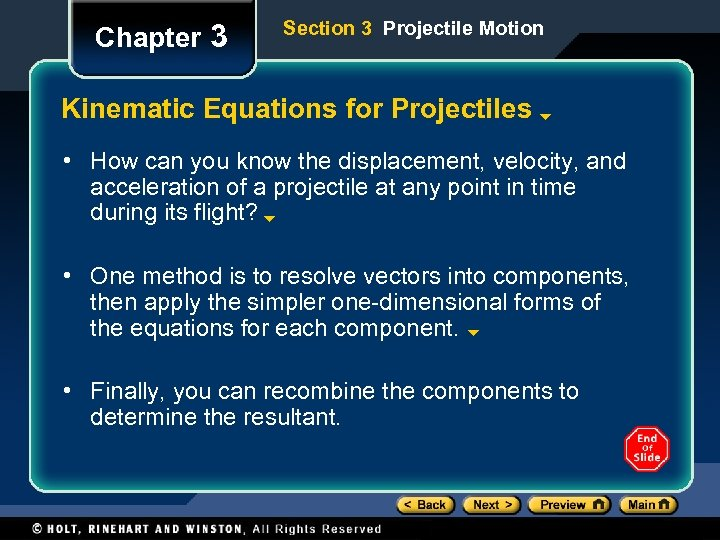Chapter 3 Section 3 Projectile Motion Kinematic Equations for Projectiles • How can you know the displacement, velocity, and acceleration of a projectile at any point in time during its flight? • One method is to resolve vectors into components, then apply the simpler one-dimensional forms of the equations for each component. • Finally, you can recombine the components to determine the resultant.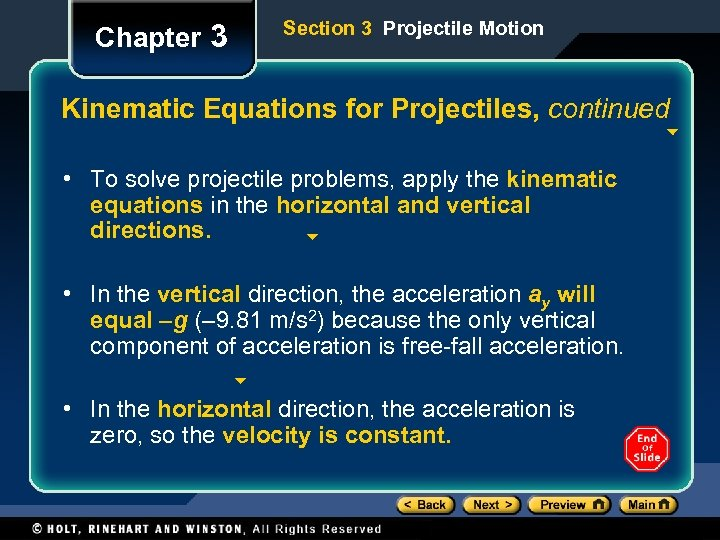Chapter 3 Section 3 Projectile Motion Kinematic Equations for Projectiles, continued • To solve projectile problems, apply the kinematic equations in the horizontal and vertical directions. • In the vertical direction, the acceleration ay will equal –g (– 9. 81 m/s 2) because the only vertical component of acceleration is free-fall acceleration. • In the horizontal direction, the acceleration is zero, so the velocity is constant.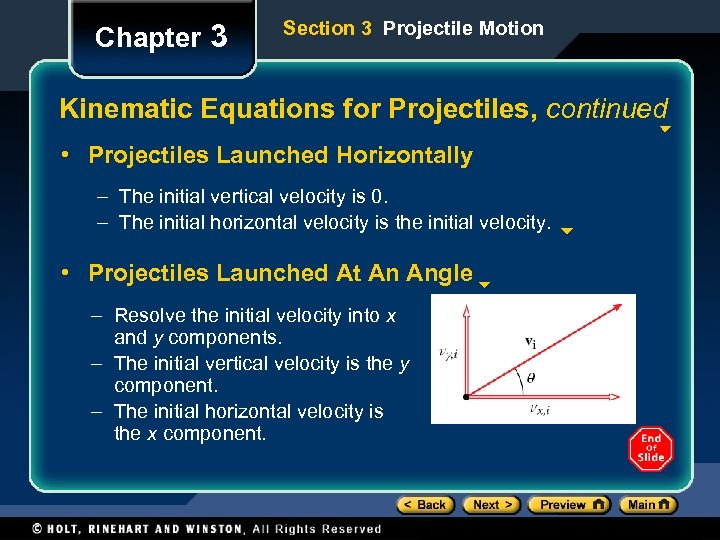Chapter 3 Section 3 Projectile Motion Kinematic Equations for Projectiles, continued • Projectiles Launched Horizontally – The initial vertical velocity is 0. – The initial horizontal velocity is the initial velocity. • Projectiles Launched At An Angle – Resolve the initial velocity into x and y components. – The initial vertical velocity is the y component. – The initial horizontal velocity is the x component.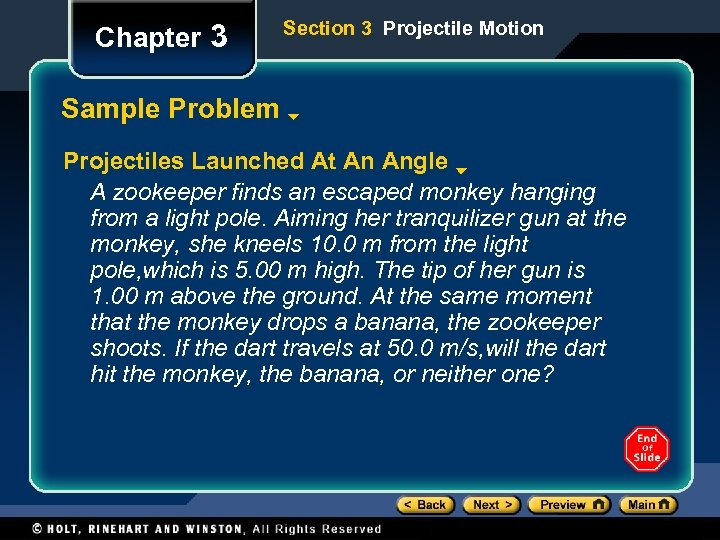Chapter 3 Section 3 Projectile Motion Sample Problem Projectiles Launched At An Angle A zookeeper finds an escaped monkey hanging from a light pole. Aiming her tranquilizer gun at the monkey, she kneels 10. 0 m from the light pole, which is 5. 00 m high. The tip of her gun is 1. 00 m above the ground. At the same moment that the monkey drops a banana, the zookeeper shoots. If the dart travels at 50. 0 m/s, will the dart hit the monkey, the banana, or neither one?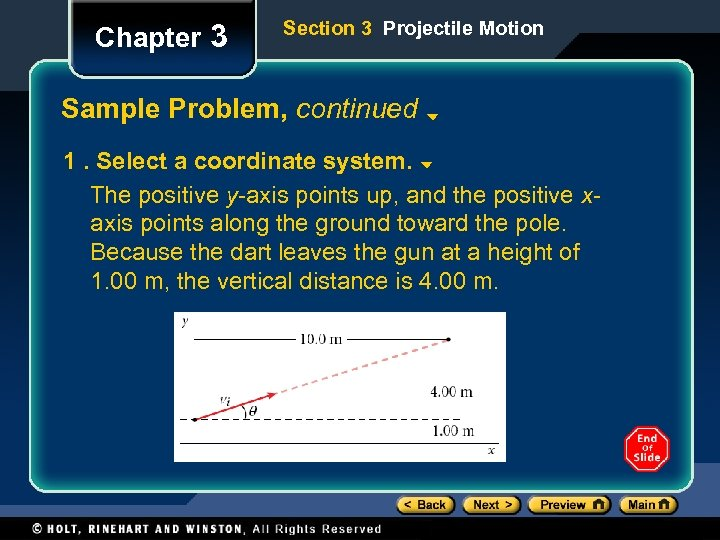Chapter 3 Section 3 Projectile Motion Sample Problem, continued 1. Select a coordinate system. The positive y-axis points up, and the positive xaxis points along the ground toward the pole. Because the dart leaves the gun at a height of 1. 00 m, the vertical distance is 4. 00 m.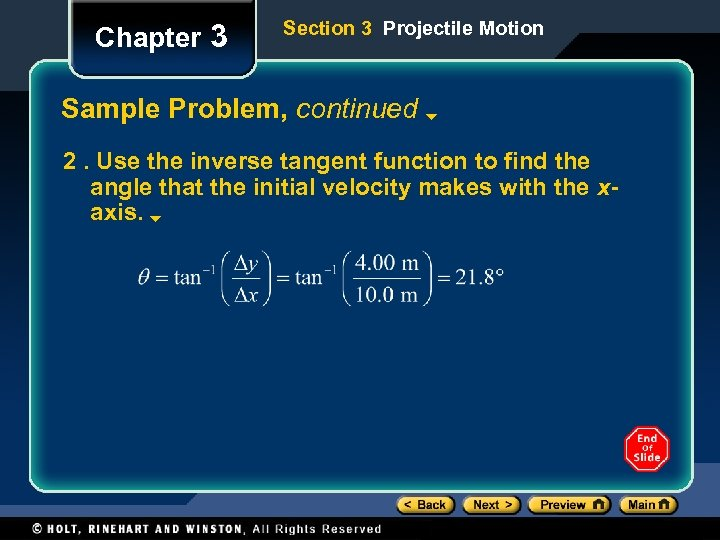Chapter 3 Section 3 Projectile Motion Sample Problem, continued 2. Use the inverse tangent function to find the angle that the initial velocity makes with the xaxis.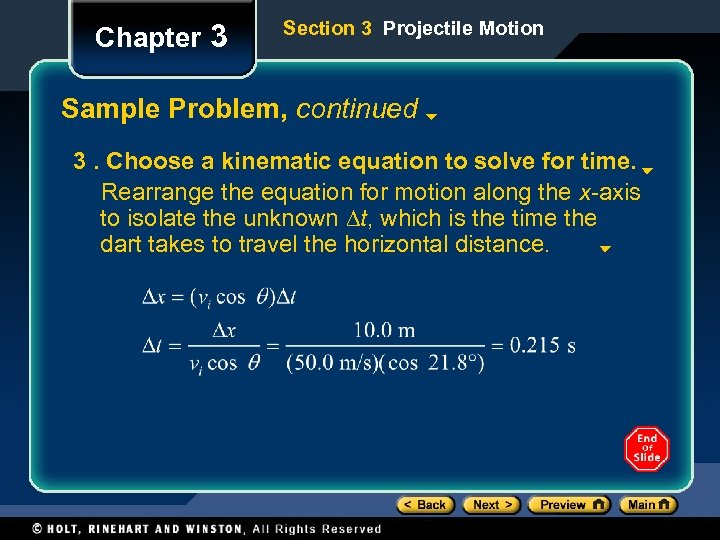Chapter 3 Section 3 Projectile Motion Sample Problem, continued 3. Choose a kinematic equation to solve for time. Rearrange the equation for motion along the x-axis to isolate the unknown Dt, which is the time the dart takes to travel the horizontal distance.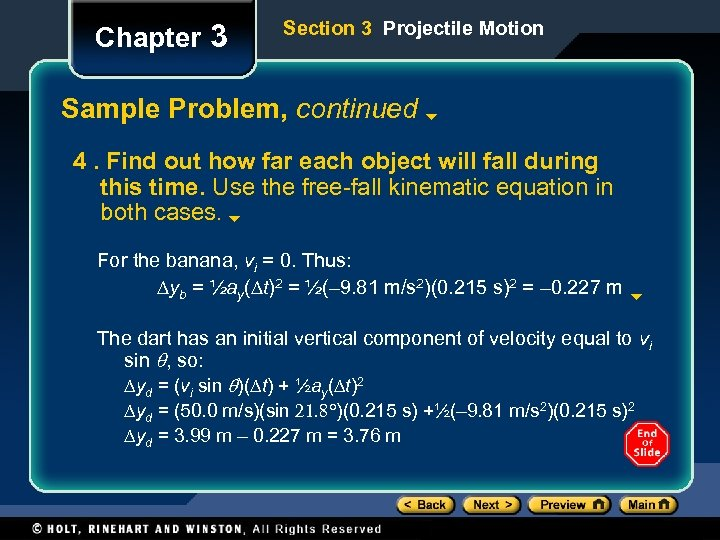Chapter 3 Section 3 Projectile Motion Sample Problem, continued 4. Find out how far each object will fall during this time. Use the free-fall kinematic equation in both cases. For the banana, vi = 0. Thus: Dyb = ½ay(Dt)2 = ½(– 9. 81 m/s 2)(0. 215 s)2 = – 0. 227 m The dart has an initial vertical component of velocity equal to vi sin q, so: Dyd = (vi sin q)(Dt) + ½ay(Dt)2 Dyd = (50. 0 m/s)(sin 21. 8 )(0. 215 s) +½(– 9. 81 m/s 2)(0. 215 s)2 Dyd = 3. 99 m – 0. 227 m = 3. 76 m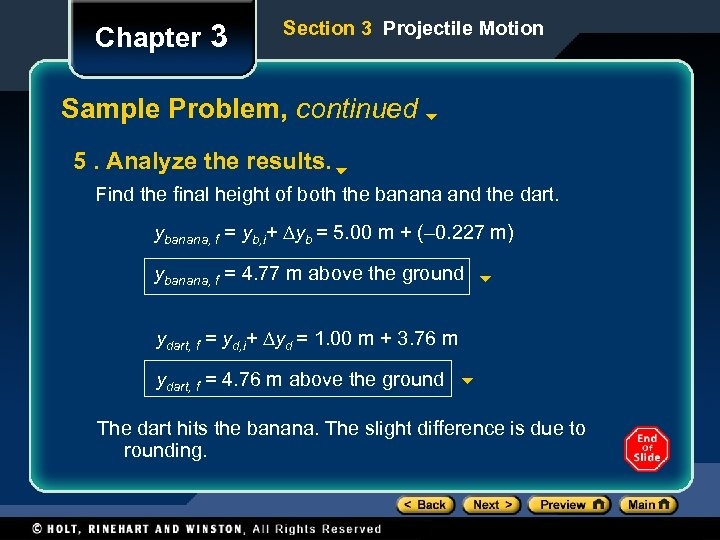Chapter 3 Section 3 Projectile Motion Sample Problem, continued 5. Analyze the results. Find the final height of both the banana and the dart. ybanana, f = yb, i+ Dyb = 5. 00 m + (– 0. 227 m) ybanana, f = 4. 77 m above the ground ydart, f = yd, i+ Dyd = 1. 00 m + 3. 76 m ydart, f = 4. 76 m above the ground The dart hits the banana. The slight difference is due to rounding.